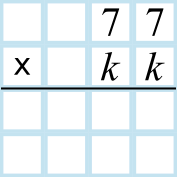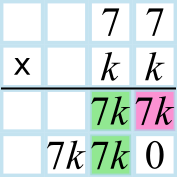#### You may also like### Consecutive Numbers

An investigation involving adding and subtracting sets of consecutive numbers. Lots to find out, lots to explore.### Calendar Capers

Choose any three by three square of dates on a calendar page...### Days and Dates

Investigate how you can work out what day of the week your birthday will be on next year, and the year after...

# Identical Digit Multiplication

##### Age 11 to 14 ShortChallenge Level

Trying out numbers
77$\times$11 = 770 + 77 = 847, which does not have a third digit of 3
77$\times$22 = 847 + 847 = 1694, which does not have a third digit of 3
77$\times$33 = 1694 + 847 = 2541, which does not have a third digit of 3
77$\times$44 = 2541 + 847 = 3388, which does not have a third digit of 3
77$\times$55 = 3388 + 847 = 4235, which does have a third digit of 3
So the product was 4235.

Multiplying 77 by a number written $kk$
Writing out the mutliplication like this,All of the mutliplications will be $7\times k$, plus carried digits and the $0$ as shown below. The third digit in the product will come from the tens digit of the pink box below added to the units digts in the green box.So the last digit of the number which is the tens digit of $7k$ plus twice the units digit of $7k$ is a $3$.
If $k=1$, then $7k=7$, and the tens digit plus twice the units digit is $0+14=14$ which has last digit 4.
If $k=2$, then $7k=14$, and the tens digit plus twice the units digit is $1+8=9$ which has last digit 9.
If $k=3$, then $7k=21$, and the tens digit plus twice the units digit is $2+2=4$ which has last digit 4.
If $k=4$, then $7k=28$, and the tens digit plus twice the units digit is $2+16=18$ which has last digit 8.
If $k=5$, then $7k=35$, and the tens digit plus twice the units digit is $3+10=13$ which has last digit 3.
So $k=5$, and the product is $4235$.

Using the 11 times table
Two-digit numbers with identical digits are precisely the multiples of 11. So 77 has been multiplied by 11$n$ for some value of $n$.

77$\times$11$n$ = (77$\times$11)$n$ = 847$n$. So 847$n$ = _ _ 3 _ .

The 800 will not contribute to this digit, so we can consider the 47 times table.
47$\times$2 = 94
47$\times$3 = 141
47$\times$4 = 188
47$\times$5 = 235
So $n$ = 5, and the product is 847$\times$5 = 4235.

You can find more short problems, arranged by curriculum topic, in our short problems collection.